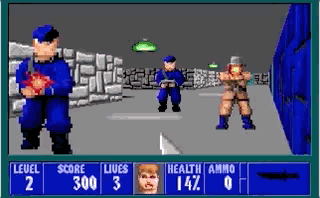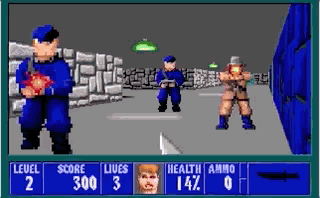### Fizzlefade Using a Feistel Network

In my last post I discussed the interesting Fizzlefade algorithm in the classic game Wolfeinstein 3D, which uses a Linear-feedback shift register to randomly iterate over all the pixels on the screen. I read another post by Antirez where he discusses using a Feistel Network as an alternative way of producing a random iteration of all pixels. This algorith can easily be scaled to any screen resolution, where the original Linear-feedback shift register was carefully chosen for the 320*200 resolution in Wolfenstein 3D.

Again, I’m going to spend some time analyzing the algorithm, just for fun. It’s rare that you come across an algorithm with such a visual application.

#### Feistel Networks

A Feistel Network or Feistel Cipher is a technique used in cryptography to construct block ciphers. In technical terms it takes an input sequence and transforms it into another sequence in such a way that the transformation is always reversible. In practice this means the Feistel Network takes a number as input and spits out another seemingly random number, but it will always yield the same output for a given input. This transformation is always reversible, which has an interesting implication - every input must have a unique corresponsing output. (If we had 2 inputs that yielded the same output we wouldn’t be able to reverse it and find the original input)

These 2 properties make a Feistel Network very useful in this scenario:

1. Every input has a unique output
2. For a given input the output is psuedorandom

Let’s go back to the original problem - we want to randomly iterate over all the pixels in a 320*200 display. `320*200 = 64000` total pixels. If we use a 16-bit integer as input that will give us `2^16 = 65536` possible inputs. By iterating over all numbers from 0 to 65535 and passing it through the the Feistel Network we will get all numbers from 0 to 65535 in a random order - we simply need to be able to take each of these numbers and plot it on a 320*200 display and we’re done! (We can ignore all results between 63999 and 65535 - there is some computational waste but it’s not significant)

#### Constructing a Feistel Network

The algorithm for constructing a Feistel Network is as follows

Split the input into 2 halves, left and right
For N rounds
next_left = right
next_right = left XOR round_function(right)
left = next_left; right = next_right
Combine left and right into a single value

So what’s the round function, and what’s the value of N? The round function is a psuedorandom random function, meaning it takes a number and spits out a different number. If it’s a very good psuedorandom function it will yield two completely different results for two very similar inputs. Here is an example of such a function (not a very good one, as we’ll see later on):

Here’s a sample of the function in action:

The Feistel Network will actually work for any function, but we want a good psuedorandom function to get the desired effect. The number N is also rather arbitrary - there is some cryptographic proof that says with an appropriate round function we can use only 5 rounds, so let’s start with 5 and see how well that works.

Now that we understand the round function and we’ve picked a value for N we can implement the Feistel Network:

We can use this Feistel Network to implement an alternative version of the fizzlefade algorithm:

(Note that I’m using the fact that a 16-bit integer will overflow and reset to 0 when I’ve iterated over all 16-bit numbers. I could have used a bigger integer, but in the spirit of the original algorithm - which was implemented in assembly - I’m trying to use the smallest amount of memory possible.)

Now here’s the really cool part: in my last post I implemented the original Fizzlefade algorithm using a Linear-feedback shift register and I wrote some tests to help me understand the algorithm. I can now swap out the original Fizzlefade implementation for this one, and if all the tests pass I know the implementation is correct!

These test will simply write to the console when a condition is not met, so when I run this I expect to see no output.

Success! What I really want though, is to be able to visualize my solution. An easy way to do that would be to use HTML Canvas, which means I need to rewrite my algorithm in JavaScript. The tricky bit is that I now need to deal with buffers - to make things easier I just calculated all the buffers in memory and then render each buffer 14 milliseconds apart. Why 14? The original game ran ran at 70 FPS (or at least tried to, since that was the maximum the graphics processing could handle) so 1000ms / 70 ~= 14.

Here’s the result:Now that we can visualize the algorithm you can see that it’s not all that random - especially towards the end of the animation you start to see patterns or ‘streaking’ towards going towards the right of the screen. Let’s see what happens if I use a better round function.

(This is the same round function that Antirez uses in his example.) Here’s the result:Much better! If you want to look at the code the C code is available on Github and the JavaScript code is on Plunker.